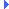FD.io VPP  v18.01-8-g0eacf49 Vector Packet ProcessingInclude dependency graph for load_balance_map.c:

Go to the source code of this file.

## Functions

static uword load_balance_map_db_hash_key_sum (hash_t *h, uword key)

static uword load_balance_map_db_hash_key_equal (hash_t *h, uword key1, uword key2)

from the paths that are usable, fill the Map. More...

static int load_balance_map_path_state_change_walk (fib_node_ptr_t *fptr, void *ctx)

the state of a path has changed (it has no doubt gone down). More...

static clib_error_tload_balance_map_show (vlib_main_t *vm, unformat_input_t *input, vlib_cli_command_t *cmd)

## Variables

static uwordlb_maps_by_path_index
A hash-table of load-balance maps by path index. More...

A hash-table of load-balance maps by set of paths. More...

The global pool of LB maps. More...

## Macro Definition Documentation

 #define LOAD_BALANCE_MAP_DBG ( _pl, _fmt, _args... )

Definition at line 81 of file load_balance_map.c.

## Enumeration Type Documentation

Enumerator

Definition at line 38 of file load_balance_map.c.

## Function Documentation

 u8* format_load_balance_map ( u8 * s, va_list * ap )

Definition at line 91 of file load_balance_map.c.Here is the call graph for this function:Here is the caller graph for this function:

Definition at line 427 of file load_balance_map.c.Here is the call graph for this function:Here is the caller graph for this function:

static

Definition at line 384 of file load_balance_map.c.Here is the caller graph for this function:

static

Definition at line 199 of file load_balance_map.c.Here is the caller graph for this function:

static

Definition at line 154 of file load_balance_map.c.Here is the call graph for this function:Here is the caller graph for this function:

 static uword load_balance_map_db_hash_key_2_index ( uword key )
inlinestatic

Definition at line 147 of file load_balance_map.c.Here is the call graph for this function:Here is the caller graph for this function:

 static uword load_balance_map_db_hash_key_equal ( hash_t * h, uword key1, uword key2 )
static

Definition at line 185 of file load_balance_map.c.Here is the call graph for this function:Here is the caller graph for this function:

 static uword load_balance_map_db_hash_key_from_index ( uword index )
inlinestatic

Definition at line 135 of file load_balance_map.c.Here is the caller graph for this function:

 static uword load_balance_map_db_hash_key_is_index ( uword key )
inlinestatic

Definition at line 141 of file load_balance_map.c.Here is the caller graph for this function:

 static uword load_balance_map_db_hash_key_sum ( hash_t * h, uword key )
static

Definition at line 174 of file load_balance_map.c.Here is the call graph for this function:Here is the caller graph for this function:

static

Definition at line 214 of file load_balance_map.c.Here is the call graph for this function:Here is the caller graph for this function:

static

Definition at line 257 of file load_balance_map.c.Here is the call graph for this function:Here is the caller graph for this function:

static

Definition at line 419 of file load_balance_map.c.Here is the caller graph for this function:

static

from the paths that are usable, fill the Map.

Definition at line 287 of file load_balance_map.c.Here is the call graph for this function:Here is the caller graph for this function:

static

Definition at line 85 of file load_balance_map.c.Here is the caller graph for this function:

static

Definition at line 118 of file load_balance_map.c.Here is the caller graph for this function:

static

Definition at line 404 of file load_balance_map.c.Here is the call graph for this function:Here is the caller graph for this function:

 void load_balance_map_lock ( index_t lbmi )

Definition at line 454 of file load_balance_map.c.Here is the call graph for this function:

Definition at line 522 of file load_balance_map.c.Here is the call graph for this function:Here is the caller graph for this function:

 void load_balance_map_path_state_change ( fib_node_index_t path_index )

the state of a path has changed (it has no doubt gone down).

This is the trigger to perform a PIC edge cutover and update the maps to exclude this path.

Definition at line 503 of file load_balance_map.c.Here is the call graph for this function:Here is the caller graph for this function:

 static int load_balance_map_path_state_change_walk ( fib_node_ptr_t * fptr, void * ctx )
static

Definition at line 485 of file load_balance_map.c.Here is the call graph for this function:Here is the caller graph for this function:

 static clib_error_t* load_balance_map_show ( vlib_main_t * vm, unformat_input_t * input, vlib_cli_command_t * cmd )
static

Definition at line 546 of file load_balance_map.c.Here is the call graph for this function:

Definition at line 537 of file load_balance_map.c.Here is the call graph for this function:Here is the caller graph for this function:

 void load_balance_map_unlock ( index_t lbmi )

Definition at line 464 of file load_balance_map.c.Here is the call graph for this function:Here is the caller graph for this function:

## Variable Documentation

 uword* lb_maps_by_path_index
static

A hash-table of load-balance maps by path index.

this provides the fast lookup of the LB map when a path goes down

Definition at line 27 of file load_balance_map.c.

static

A hash-table of load-balance maps by set of paths.

This provides the LB map sharing. LB maps do not necessarily use all the paths in the list, since the entry that is requesting the map, may not have an out-going label for each of the paths.

Definition at line 36 of file load_balance_map.c.

The global pool of LB maps.

The encapsulation breakages are for fast DP access.

Definition at line 69 of file load_balance_map.c.